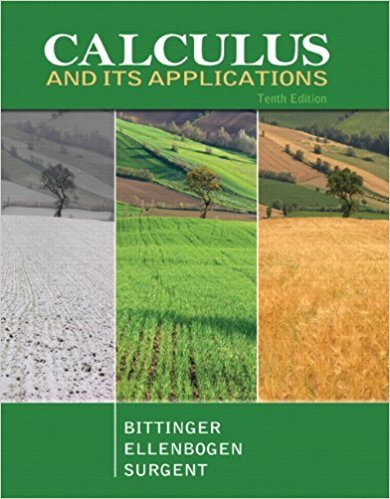×
×

# Solutions for Chapter 6.2: Partial Derivatives## Full solutions for Calculus and Its Applications | 10th Edition

ISBN: 9780321694331Solutions for Chapter 6.2: Partial Derivatives

Solutions for Chapter 6.2
4 5 0 337 Reviews
31
4
##### ISBN: 9780321694331

Since 69 problems in chapter 6.2: Partial Derivatives have been answered, more than 25126 students have viewed full step-by-step solutions from this chapter. This textbook survival guide was created for the textbook: Calculus and Its Applications, edition: 10. This expansive textbook survival guide covers the following chapters and their solutions. Chapter 6.2: Partial Derivatives includes 69 full step-by-step solutions. Calculus and Its Applications was written by and is associated to the ISBN: 9780321694331.

Key Calculus Terms and definitions covered in this textbook
• Absolute value of a complex number

The absolute value of the complex number z = a + b is given by ?a2+b2; also, the length of the segment from the origin to z in the complex plane.

P(A or B) = P(A) + P(B) - P(A and B). If A and B are mutually exclusive events, then P(A or B) = P(A) + P(B)

• Angle of depression

The acute angle formed by the line of sight (downward) and the horizontal

• Degree

Unit of measurement (represented by the symbol ) for angles or arcs, equal to 1/360 of a complete revolution

• Elements of a matrix

See Matrix element.

• Equilibrium price

See Equilibrium point.

• Explanatory variable

A variable that affects a response variable.

• Horizontal component

See Component form of a vector.

• Intercept

Point where a curve crosses the x-, y-, or z-axis in a graph.

See Polynomial function in x

• Octants

The eight regions of space determined by the coordinate planes.

• Probability of an event in a finite sample space of equally likely outcomes

The number of outcomes in the event divided by the number of outcomes in the sample space.

• Product of complex numbers

(a + bi)(c + di) = (ac - bd) + (ad + bc)i

• Product rule of logarithms

ogb 1RS2 = logb R + logb S, R > 0, S > 0,

• Reflection through the origin

x, y and (-x,-y) are reflections of each other through the origin.

• Scientific notation

A positive number written as c x 10m, where 1 ? c < 10 and m is an integer.

• Slant asymptote

An end behavior asymptote that is a slant line

• Standard form of a polar equation of a conic

r = ke 1 e cos ? or r = ke 1 e sin ? ,

• Variable (in statistics)

A characteristic of individuals that is being identified or measured.

• Weighted mean

A mean calculated in such a way that some elements of the data set have higher weights (that is, are counted more strongly in determining the mean) than others.

×